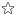quizzes  Forums# Bases and Exponents

created by Simmy8 on 17 Aug 12Medium level (85% of success)    12 questions - 143 players
Evaluate Expressions1
Find the value of 36^2

2
Find the value of 5^3

3
What is 70 * 70 expressed as a single base with an exponent?

4
What is 20 * 20 * 20 expressed as a single base with an exponent?

5
What is 8^3 ?

6
What is 7^2 ?

7
What is a way to solve 43^3 ?

8
What is a way to solve 24^2?

9
What is 5^2

10
Which expression shows 67^3 by using repeated multiplication?

11
Which expression shows 77^2 by using repeated multiplication?

12
What is the exponent of the expression 14^2?

Quiz.biz does not certify the quizzes to be reliable, contact Simmy8 !Comment this Quiz
If you want to rank and comment this quizz, login in or create an account !